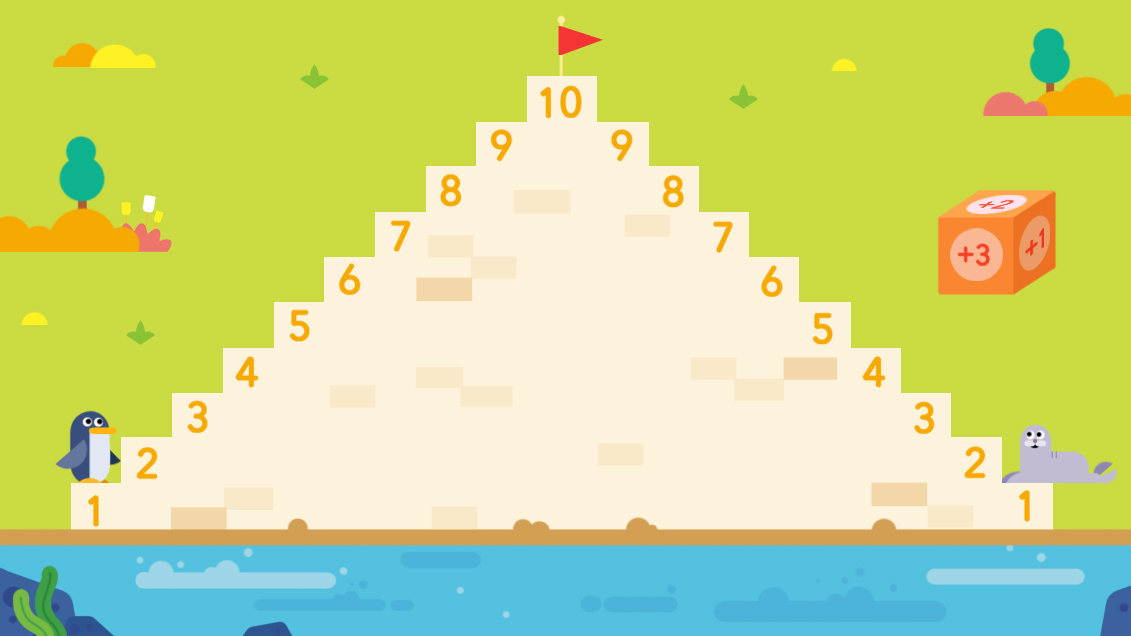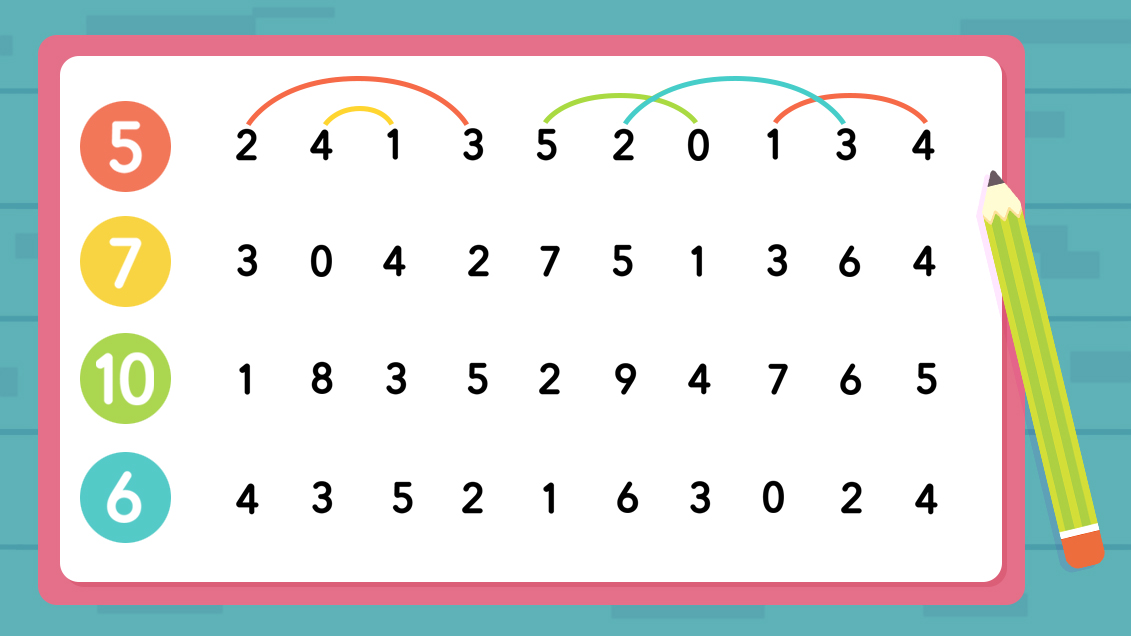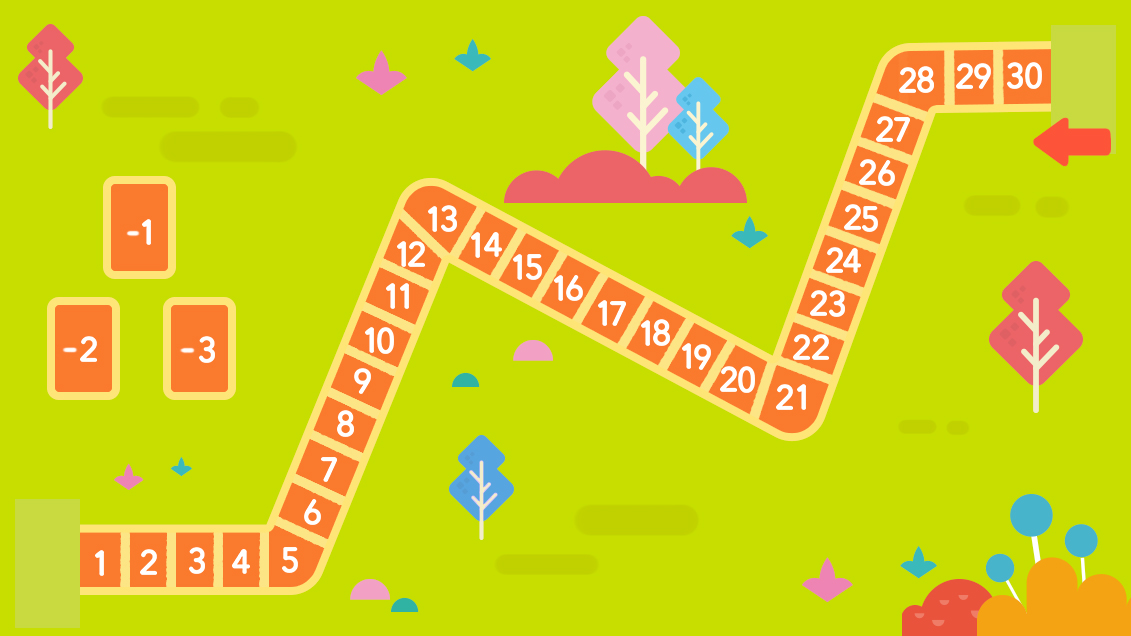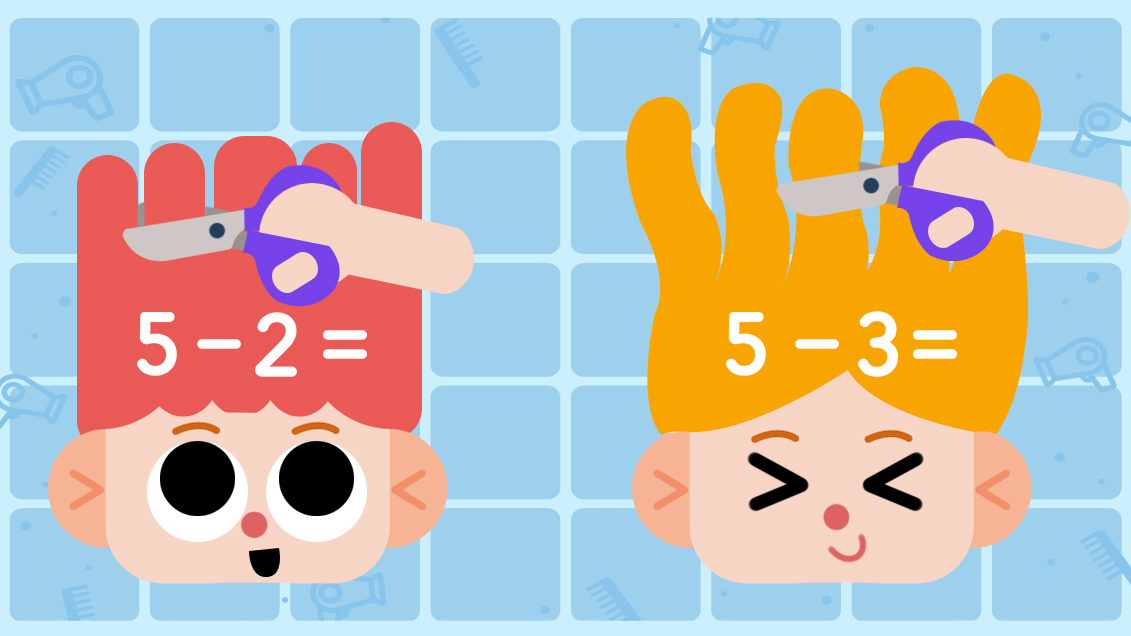# Put Some Fun in the Fundamentals: Numbers Added and Subtracted

by Tom, Jun 22 2022

Your child is growing up and starting to learn those essential math skills - learning how numbers are added together and subtracted, maybe even 'part and whole' combination and division. Mastering addition and subtraction is a process and, as your child develops, they'll advance from counting aloud numbers one by one to quickly reaching the answer through mental math.

If your child is struggling to make that next step, though, we've got you covered with these four fun games you can play together. Teach your little one Building up their confidence and their skills is the cornerstone for a lifetime of success. Let's get into it!

## 1. Sums to the Summit

This game is a great way to practice those addition skills. First, take a piece of paper and draw a staircase with ten stairs. Number each stair from one to ten. Then you'll need to make the Addition Die, a folded cube of paper. Write +1, +2, and +3 on the faces, making sure each is used twice. Finally, grab two small objects to use as playing pieces.

Each player starts at 1 on the staircase and rolls the Addition Die. The numbers on each will be added together. Add the number on the Die to the stair you're on, say the result, and move your piece up the stairs. For example, "1 plus 2 is 3," and move to the third stair. Then it's the other player's turn. The first player to reach the summit at number 10 is the winner.## 2. Let's Connect

This is another game to help children practice their sums. You'll need a sheet of paper and a pen or pencil. First, take your paper and write a number between 5 and 10 on the left side. To the right of that number, write smaller numbers that can be added together to make the number on the left. Let's look at the example below.The big numbers on the left are 5, 7, 10, and 6. The rows of numbers to the right of them can be added together to reach that number.

Play proceeds by using your pencil to connect the small numbers in the right row that sum to reach the big number on the left. In the above example, 7 can be made of 3 and 4, 7 and 0, and so on.

## 3. Race to One

Now let's look at two games to practice subtraction skills. For this game you'll need a few pieces of paper, something to write with, and two small items to use as playing pieces. Start by drawing a road on one sheet of paper, and segment it into numbered blocks. Then make your playing cards by cutting another sheet of paper into card-sized squares and writing -1, -2, and -3 on them. Put them face down nearby. Finally, put your game pieces on a starting number - we recommend starting at 30.

Players will take turns drawing a card and subtracting its value from the square their piece is standing on. For example, if my piece is at 30 and I draw -2, I should say, "30 minus 2 is 28," and move down to square 28. The winner is the first player to reach the number 1 square.## 4. Happy Haircuts

For this game, grab a few sticky notes, a pair of scissors, and a single sheet of paper. Let your child draw two funny faces on the paper while you work on the sticky notes. Cut a little more than halfway down the length of each sticky note to make 'hair.' Then write a subtraction problem at the base of the note based on how much 'hair' the note has. In the example photo below, the note on the left has 5 strands of hair and the problem is 5-2.Guide your child to cut as many pieces of hair from the note as the equation tells them to. Help them to say the equation, too: 'five minus two is three.' This funny game is a hands-on activity that brings laughter to learning while your child bridges the gap between abstract equations and concrete operations.